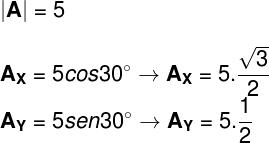Mechanics

# Vectors

Vectors are line segments used to represent some vector quantity.

A vector is an oriented line segment that has modulus (size), direction, and direction. Vectors are used to express vector physical quantities , that is, those that can only be completely defined if we know their numerical value, the direction in which they act (horizontal and vertical), as well as their direction (up, down ).

Position, velocity, acceleration, force, and momentum are good examples of vector quantities . For example, if we want to know the position of a location, it is necessary to point it in a direction. In this case, the direction of movement is given by the fingertip.

In order to draw vectors, it is necessary to realize that their representation must take into account their size, that is, a vector that represents a quantity with a numerical value equal to 10 must be drawn with half the size of a vector that has size 20.

The size at which we draw a vector represents its module.

The directions of a vector can be defined based on the chosen coordinate system , for example. Using the Cartesian system , the directions of space would be x and y and a vector could be written as V = (x, y). The direction, in turn, concerns the arrow at the tip of the vector, which indicates it, and can be either positive or negative.

When we write that a vector is defined by its x and y coordinates, we say that x and y are its horizontal and vertical components, respectively. When a vector is inclined, without coinciding with any of the axes of the coordinate system, it is possible to determine the size of its components . To do so, it is enough to know the angle θ, formed between the vector and the horizontal direction, and the magnitude of the vector a:

To calculate these components, it is necessary to do the following calculation: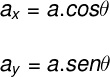Based on the x and y components of a vector, it is possible to calculate its magnitude (size). For this, we simply apply the Pythagorean theorem , since these components are perpendicular to each other: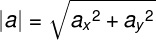## Result vector

Result vector is the name given to the vector obtained after performing a vector sum . In the vector sum, we must consider the magnitude , the direction and the direction of the vectors to find the resulting vector. Here are some cases of operations with vectors.

## vector operations

### → Sum of vectors

Parallel vectors are those that meet in the same direction and in the same direction. The angle formed between these vectors is always zero. Look at the figure below:

If these vectors also have the same magnitude, we say that they are equal vectors. To find the resultant of these vectors, just add the modulus of each one, in addition, the resulting vector will be in the same direction as the parallel vectors, and its size should be the size of the two original vectors:

To calculate the magnitude of the vector R , we can use the following formula: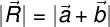### → Subtraction of vectors

Opposite vectors make an angle of 180º to each other, meet in the same direction, but with opposite directions, as shown in the figure:

The resulting vector of two opposing vectors is given by the difference in their magnitude, as you can see in the following figure:

In this case, the resulting vector will have its direction and direction determined by the vector with the highest magnitude and can be calculated using the following formula: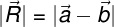### → Perpendicular vectors: Pythagorean Theorem

Perpendicular vectors form a 90° angle to each other. To find the vector resulting from two perpendicular vectors, we must connect the beginning of one of the vectors to the tip of the other. The resulting vector, in this case, will form the hypotenuse of a right triangle, note:

The magnitude of this resulting vector can be calculated using the Pythagorean theorem: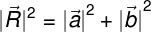### → Oblique vectors: parallelogram rule

Vectors that do not fit in any of the previous cases can be determined geometrically by the parallelogram rule, as in the next figure:

Since θ is the angle formed between the two base vectors (blue and red), the magnitude of the resulting vector can be obtained through the following formula: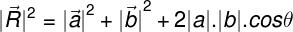### → Result of several vectors

When we have several vectors and we want to find the resulting vector, we must connect them to each other. In this process, which does not depend on the order chosen, we must connect the tip of one vector to the beginning of the next. In the end, the resulting vector will be the one that connects the beginning of the first vector with the tip of the last one:

To find the magnitude of this vector, we add the x and y components of each of the vectors a , b , c , and d , and in the end we apply the Pythagorean Theorem.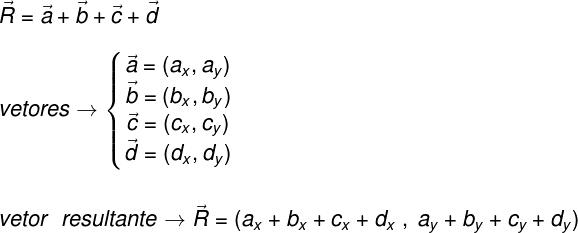## Exercises solved

1 ) Mark the alternative that correctly presents the magnitude of the resultant of two vectors, A and B , whose components are given by A = (12,5) and B = (-9,-1).

a) 12

b) 4

c) 6

d) 5

e) 3

Template: Letter D

Resolution:

To determine the vector resulting from vectors A and B , we need to add their x and y components, for that, we will do the following calculation: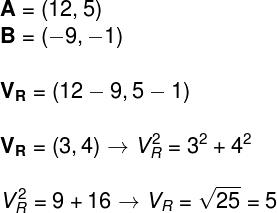According to the result found, the resulting vector is given R = (3,4) and its modulus is 5.

2) Two vectors, of magnitudes 3 and 2, form an angle of 60º with each other. Determine the magnitude of the resultant of these vectors.

a) 6

b) √6

c) 5

d) √19

e) 5

Template: Letter E

Resolution:

To calculate the magnitude of the resulting vector between these two oblique vectors, it is necessary to use the law of cosines, considering that the angle between these vectors is 60º. Therefore, we will have to do the following calculation: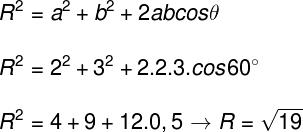3) A vector A, of magnitude 5, is inclined at an angle of 30º in relation to the horizontal axis. Determine the magnitude of the horizontal and vertical components, Ax and Ay , of these vectors.

a) √3 and √2

b) 5√3/2 and 5/2

c) 5/2 and 5

d) 3/4 and 5/2

e) 25 and √2

Template: letter B

Resolution:

To determine which are the components of vector A , we must use the relations of its modulus with the sine and cosine of the 30º angle, which this vector forms with the x direction. To do so, we must perform the following calculation: